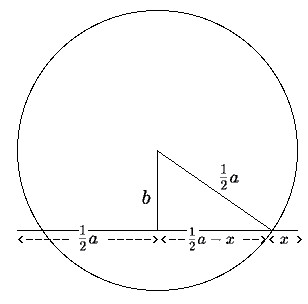# Euclid's geometric solution of a quadratic equationWe find $x$ so that $(a - x)x = b^{2}$.

Draw a line of length $a$.

Draw a line of length $b$ perpendicular to the line $a$ from its midpoint.

Draw a circle centred at the end of the line with radius $\large\frac{1}{2}\normalsize a$.

Now $x$ is a solution of $(a - x)x = b^{2}$.

To see this use the fact that $(\large\frac{1}{2}\normalsize a)^{2} -(\large\frac{1}{2}\normalsize a - x)^{2} = b^{2}$.

But $(\large\frac{1}{2}\normalsize a)^{2} - (\large\frac{1}{2}\normalsize a - x)^{2} = (a - x)x$ so $x$ is the required solution.